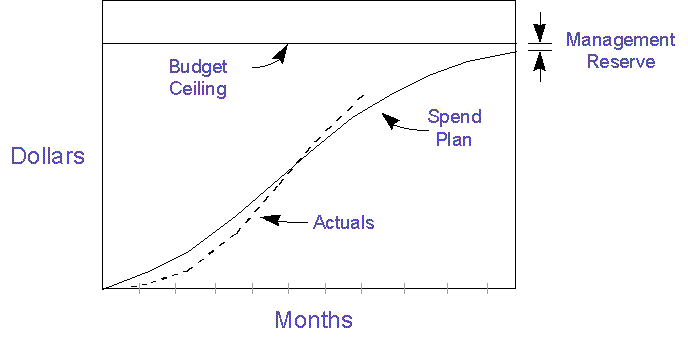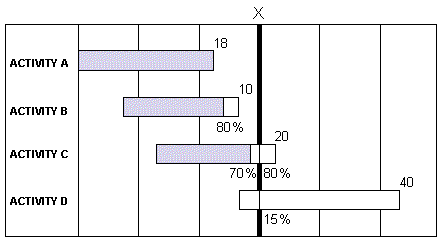Earned Value Management

Project Budget:

The most basic cost control technique is to develop a project budget and then track spending against it.  On a small project, this can be as simple as having a target cost goal for the total project. You could monitor project costs and sound the alarm if the percent of dollars spent exceeds the percent completion estimated for the project. You could also prepare a time-phased budget, as shown in the figure below, breaking the overall budget goal into intervals of weeks, months, quarters or years. This can provide a budget baseline for tracking actual costs against periodic budget targets. When the cumulative budget of estimated project costs are plotted graphically over time, they usually result in the shape illustrated, which is sometimes called an "S" curve, since it looks like an inclined "S."

Budget Spend Plan Tracking:

A simple technique for tracking project costs is to develop a weekly or monthly cumulative budget spend plan and then track actual costs against the plan. The slope of the spend plan indicates the project expenditure rate, sometimes called the "burn rate." By plotting actual costs against the budget spend plan, you can see differences between actual spending and the spend plan. This technique provides a simple, top-level view of project financial performance that can be useful for executive briefings, especially where you want to match expenditures to a funding stream.

Budget Spend PlanThe spend plan can be generated using a project management software tool. If you are using Microsoft Project, you can copy the hours from the Resource Usage table into MS EXCEL, apply labor rates to each resource type, and then sum costs by week to provide an accurate resource loading profile and spend plan. This can also be used to establish a performance measurement baseline of the Budgeted Cost of Work Scheduled. (See sections below on Earned Value Management Systems.)

If the project is on schedule, the spend plan method provides the needed budget status information. If the project shown were behind schedule, the project manager would no longer be able to understand project status from this graph. The budget picture would be worse than it looks, but it would be impossible to quantify.

When a project is sufficiently large or complex that it is unclear which project elements are contributing to deviations from the budget plan, a more rigorous approach to cost and schedule tracking should be employed. This following method links cost and schedule performance together and presents them in a form that facilitates management analysis and presentation.

Earned Value Management System (EVMS):

Project cost and schedule performance measurements should really be managed as integrated elements and not as separate entities.  If your budget spend plan shows you over spending and your schedule shows milestones slipping, you can know you may be in trouble, but you will have no way to make a quantitative assessment of how bad the trouble is.  EVMS solves this problem by providing an accurate picture of spending and accomplishments related to a baseline plan.  This enables you to quickly form conclusions about the project team's staffing levels and productivity, as well as giving insight into areas of the WBS where the problems are occurring.  I will never run a project without at least applying EVM principles if not having an informal or formal EVM tracking system in place.

In the past, EVM has been called Cost/Schedule Control System (C/SCS) or, for the old-timers, 7000.2 or C-Spec, after the DoD standards that originated the approach. Earned Value Management provide an integrated view of cost and schedule performance.   Unless you are tracking earned value, you really have no idea what is going on with your project!

Understanding the Basics of EVM:

EVM compares three pieces of information:

• How much work you planned to have accomplished by now (in dollars or hours) called the Planned Value;

• How much you have actually spent by now (in dollars or hours), called Actual Cost; and

• The value, in terms of your baseline budget, of the work accomplished by now (in dollars or hours), called the Earned Value!

The first two pieces of data are compared to the Earned Value in terms of differences and ratios, to result in variances and performance indexes.  That is the essence of EVM; the rest is details.

Thinking of EVM calculations in terms of remembering formulas, or for you Latin scholars - formulae, is a way to make things more complicated and obscure, although some may find it comforting to memorize formulas until they understand.  If you understand the following explanation, you will not need to think in terms of formulas.

The thing to remember is that Earned Value is the new kid on the block, so all the basic calculations involve differences or ratios with respect to Earned Value.

The difference between Earned Value and your plan (PV) is Schedule Variance,

The difference between Earned Value and your spending (AC) is Cost Variance,

The ratio of Earned Value to plan (PV) is your Schedule Performance Index,

The ratio of Earned Value to cost (AC) is your Cost Performance Index.

You can figure out what is subtracted from what by remembering that positive variance is favorable (good) and negative is unfavorable (bad).

You can figure out what is on top of an EVM ratio, by remembering that >1 is favorable and <1 is unfavorable.

So much for the basic formulae!

Interpreting EVM Computations:

• When you take a ratio of what you have done by now (EV) to what you have spent (AC), you get a reading on productivity called a cost performance index (CPI).

• When you compare where you are by now (EV) with where you planned to be (PV), you get a reading of progress called the schedule performance index(SPI).

• If instead of ratios, you take the differences, then they are called cost and schedule variances.

You can look at these cumulative to date or for the last period.  You can also look at these at the total project level and for lower levels of the WBS.

• If you have good productivity and slow progress, then you are understaffed.

• If you have low productivity, then either you have too much unplanned work or you have estimated poorly and the project has more work content than you thought.

Earned Value Implementation (Informal Approach)

To determine earned-value performance measurements for your project, you need to accomplish four steps:

1. Establish a performance measurement baseline in dollars (or hours) (this will determine the "budgeted cost of work scheduled" for the life of the project);

2. Determine "earned value" for work accomplished to date (earned value is the "budgeted cost of the work performed");

3. Relate earned value to the budgeted cost of work scheduled; and

4. Relate "actual cost of work performed" to earned value.

These four steps provide data to present a comprehensive picture of project cost and schedule performance.

Implementation of such a process can be scaled to fit projects of varied size and complexity. One should select the appropriate level of the WBS for data gathering and appropriate means for determining earned value. This can range from a few top-level categories where earned-value is determined from percent completion estimates to projects where earned value is determination is preplanned based on assignment of dollar values to completion of specific milestones or subtasks within tasks.

When the project schedule is expressed as budgeted dollars (or hours of work), you can determine the Budgeted Cost of the Work Scheduled (BCWS) at any point. This involves determining the amount of work that was scheduled to have been accomplished under the baseline plan. (For large projects, near-term WBS elements can be budgeted into work-packages, while future tasks may have budget values allocated as future planning packages or as undistributed budget.)

After the performance measurement baseline (the Budgeted Cost of Work Scheduled) is determined, project status should be evaluated to assess earned value for tasks in progress and completed. Rules for determining earned value for each work package may be established during initial cost/schedule control system implementation. Earned value can be determined by such methods as:

• pro-rating value based on task percent completion;

• linking earned value to sub-task completions, where a predetermined amount is accrued for each completed sub-task;

• earning value for draft, final, and approval of a project document; or

• accruing part of earned value at successful completion of test cases.

Summing earned value across the WBS determines the Budgeted Cost of the Work Performed. If the work is on schedule, the BCWS will be equal the BCWP (if the earned value accurately reflects performance). If the project cost actuals are on target, the Budgeted Cost of the Work Performed will be equal to the Actual Cost of the Work Performed.

Earned Value Management Example:The figure above illustrates how to determine the Budgeted Cost of Work Scheduled by summing the dollar values of the work scheduled for accomplishment at the end of period "X". The Budgeted Cost of Work Performed is determined by summing the earned value for the work actually accomplished, shown in gray shading.

PLANNED VALUE (Budgeted cost of the work scheduled) = 18 + 10 + 16 + 6 = \$50

EARNED VALUE (Budgeted cost of the work performed) = 18 + 8 + 14 + 0 = \$40

ACTUAL COST (of the work performed) = \$45 (Data from Acct. System)

Therefore:

Schedule Variance = 40 - 50 = -\$10

Schedule Performance Index = 40 / 50 = 0.8

The above example illustrates the concept of determining the Planned Value by summing the dollar values of the work scheduled for accomplishment at the end of period "X". The Earned Value is determined by summing the budgeted value for the work actually accomplished, shown in gray shading.

The Cost Variance is the difference between the Earned Value and the Actual Cost. In the example above, we are pretending our accounting system gives us "actuals" of \$45, so there is a \$5 negative cost variance. The Cost Performance Index (CPI) is 40/45 = .89. That means the project is spending about \$1.13 for every \$1.00 of (EV) budgeted work accomplished.

Projections Based On EVM Data:

One can make a rough approximation of schedule slippage using the project spend rate and the Schedule Variance, but such a method is not considered technically valid. If the spend rate is \$200K per month and the SV is \$300K, you might want to say the project is 1.5 months behind, but that would be a rough approximation.

EVM data is often used for projecting an Estimate-at-Completion (EAC).  There are various methods of doing this, and it is probably best to use a few different methods to determine a range of EACs before picking a number that looks most probable.

The simplest is to take the original cost estimate for the project, called the Budget-at-Completion, and divide that by the Cost Performance Index.  If the BAC is \$1,000,000 and the CPI is 0.9, you have reasonable grounds for an EAC of 1M/.9 or \$1.111M.

When you are behind schedule, Schedule Variance often turns into Cost Variance, especially if there is pressure to achieve the original schedule goal.  Based on this, a somewhat pessimistic EAC can be computed as BAC/ (CPI*SPI), which would reflect the compound impact of schedule and cost problems on the project.

If you have grounds for believing that productivity or staffing problems have been corrected, you could compute a Cost-to-Complete, using a more favorable CPI, and then append that to the Actual Cost.  This would have to be considered a Rosy Scenario approach.